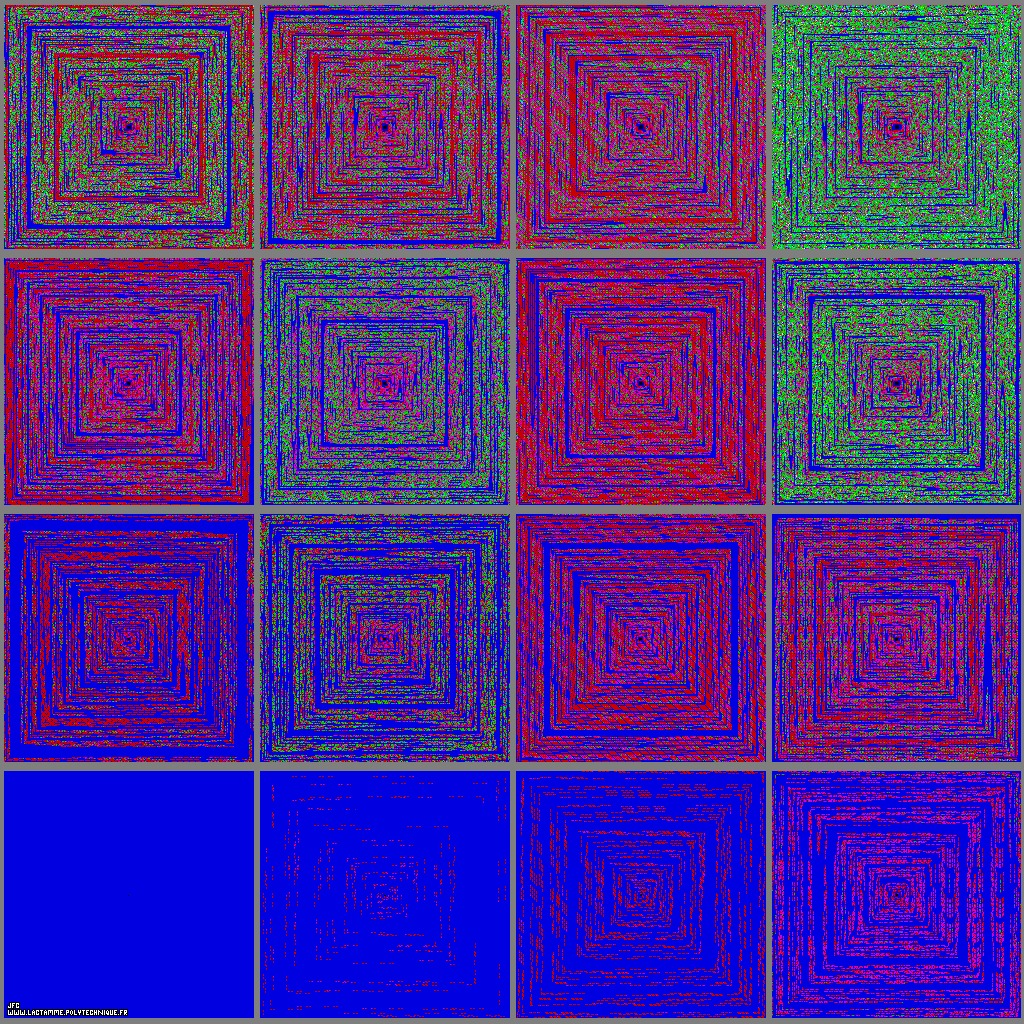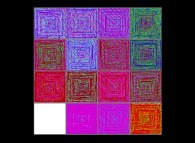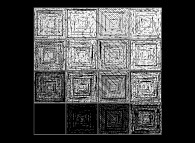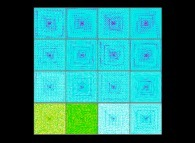The multiplicative persistence of the 65536 first integer numbers for the bases 2 -lower left- to 17 -upper right- [La persistance multiplicative des 65536 premiers nombres entiers pour les bases 2 -en bas et à gauche- à 17 -en haut et à droite-].

• Starting from the origin of the coordinates (at the center of the picture), one follows a square spiral-like path and numbers each integer point encountered (1, 2, 3,...):

```                    5----4----3
|         |    .
|         |    .
6    1----2    .
|              |
|              |
7----8----9----10
```

• Then one displays the N-th point with the false color f(PM(N,B)) where:
```                    PM(N,B) = the multiplicative persistence of N for the base B,
f(...)  = an arbitrary ascending function.
```
Let's define PM(N,B) with an obvious example:
```                    B = 10
1      0
N = 77 (= 7xB  + 7xB )
```
Then the following sequence is computed:

## ``` 77 ---> (7x7) = 49 ---> (4x9) = 36 ---> (3x6) = 18 ---> (1x8) = 8 1 2 3 4 ```

It takes four (4) steps to reach a one digit number (by the way it is the longest sequence with a two digit number). Then:
```                    PM(77,10) = 4
```

A conjecture states that PM(N,10) cannot exceed 11...

Here is an example of a longer sequence:

## ``` 48699984 ---> 4478976 ---> 338688 ---> 27648 ---> 2688 ---> 768 ---> 336 ---> 54 ---> 20 ---> 0 1 2 3 4 5 6 7 8 9 ```

• More information on this subject is available in the august 2013 issue of PLS (Pour La Science) with the Jean-Paul Delahaye's paper La persistance des nombres.

In this set of 4x4 pictures -with global renormalization- only the maximum of the sixteen maxima of PM(N,B) (B={2,3,...,16,17}) is displayed as a white point (in fact too small to be seen).

See some related pictures:See some pictures related to the additive persistence:(CMAP28 WWW site: this page was created on 05/31/2013 and last updated on 04/19/2019 14:57:08 -CEST-)

[See all related pictures (including this one) [Voir toutes les images associées (incluant celle-ci)]]

[Go back to AVirtualMachineForExploringSpaceTimeAndBeyond [Retour à AVirtualMachineForExploringSpaceTimeAndBeyond]]
[The Y2K bug [Le bug de l'an 2000]]

[Site Map, Help and Search [Plan du Site, Aide et Recherche]]
[Mail [Courrier]]
[About Pictures and Animations [A Propos des Images et des Animations]]